• 区域生长连通区域计算曲线的断点查询实验背景 勾画最内圈水纹 一 区域生长 函数输入 f 原图像输入 S可以是一个数组，也可以是一个数字，若S是一个标量，则f中有着该值的所有点都将变成种子。 T可以是一个...
区域生长

连通区域计算

曲线的断点查询

实验背景 勾画最内圈水纹 一 区域生长 函数输入
f 原图像输入
S可以是一个数组，也可以是一个数字，若S是一个标量，则f中有着该值的所有点都将变成种子。
T可以是一个数组，也可以是一个标量，若T是一个标量，则它会定义一个全局阈值。阈值用来检测图像中的像素是否与该种子或8连接种子足够相似。 函数输出
g是分割后的图像，每个区域的图像都用整数标出。
NR是不同区域的数目。
TI是一幅包含种子点的图像，该图中包含经过连通区域处理前通过阈值测试的像素。
SI和TI的大小与f相同。

function [g, NR, SI, TI] = regiongrow(f, S, T)
f = double(f);
if numel(S) == 1   %如果只给了一个种子值，也就是说 像素为这个值的点为种子点。
SI = f == S;    %SI是种子点的图像，种子点对应1，其他对应0
S1 = S;         %S1是种子值
else
SI = bwmorph(S, 'shrink', Inf);    %n = Inf时，将目标缩成一个点。没有孔洞的目标缩成一个点，有孔洞的目标缩成一个连通环。
J = find(SI);
S1 = f(J); % Array of seed values.
end

TI = false(size(f));                 %创建逻辑全0矩阵
for K = 1:length(S1)                 %将f每一个像素点依次与所有种子点的值比较，如果差值在T以内，TI对应的地方为1
seedvalue = S1(K);
S = abs(f - seedvalue) <= T;
TI = TI | S;                      %TI是由F中满足阈值测试的像素组成的图像，满足的为1，不满足的为0
end

[g, NR] = bwlabel(imreconstruct(SI, TI));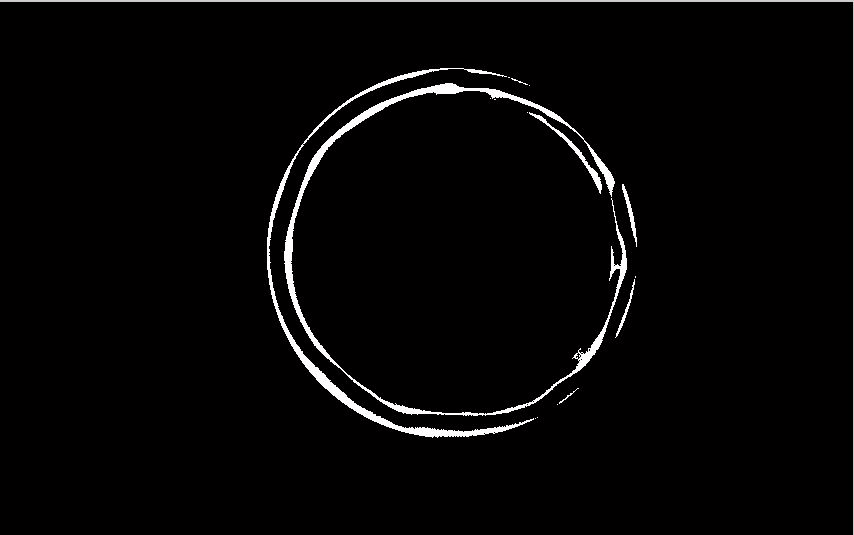二 分区域区域生长

x_start=1670;%起始点坐标
y_start=820;
x_end=2270;%终止点坐标
y_end=1220;
X=4000;
T=2400;
g(y_start:y_end,x_start:x_end)=regiongrow(tem(y_start:y_end,x_start:x_end), 3000, 500);
figure(229)
imshow(g);

三 连通区域计算

imBw = im2bw(I);                        %转换为二值化图像
imLabel = bwlabel(imBw);                %对各连通域进行标记
stats = regionprops(imLabel,'Area');    %求各连通域的大小
area = cat(1,stats.Area);
index = find(area == max(area));        %求最大连通域的索引
%idx = find([stats.Area] > 2800);       %选取特定像素大小的连通区域
img = ismember(imLabel,index);          %获取最大连通域图像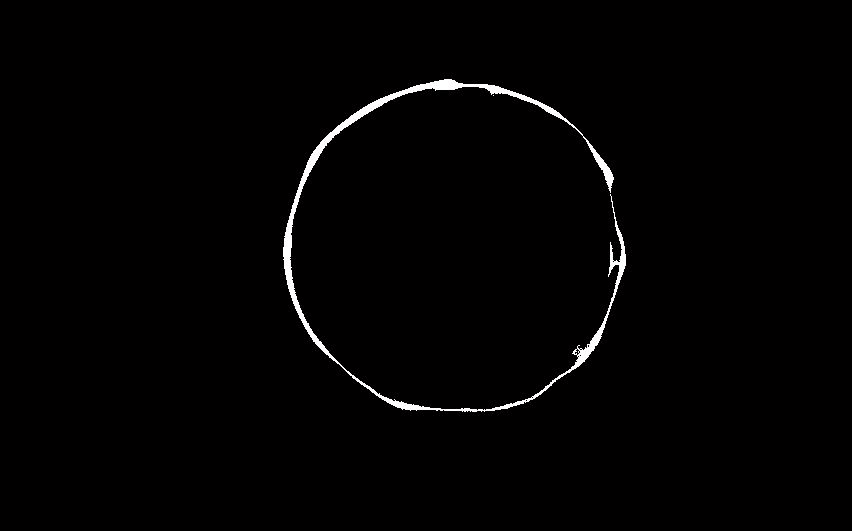四 曲线的断点查询

function g = endpoints(f)
persistent lut
if isempty(lut)
lut = makelut(@endpoint_fcn,3);
end
g =applylut(f,lut);

function is_end_point = endpoint_fcn(nhood)
is_end_point = nhood(2,2) &(sum(nhood(:)) == 2); %nhood(2,2)指的是3*3模板中中心位置的点，其坐
%标为（2，2）。这个逻辑表达式指：3*3的模板nhood
%的中心位置（2，2）位置的值为1，且和等于2.
展开全文连通域分析 曲线
• 检测图片连通区域计算物体个数，采用标签传递算法，matlab实现
• 1、Matlab 代码 %% 圆度的计算 % 圆形需要是白色的，背景是黑色的，可以根据需要确定是否对图像进行反向 clc clear;close all; % %读取源图像 I = imread('1.bmp'); % figure;imshow(I); % %灰度化、取反 h = ...
1、Matlab
代码
%% 圆度的计算
% 圆形需要是白色的，背景是黑色的，可以根据需要确定是否对图像进行反向
clc
clear;close all;
%
%读取源图像
I = imread('1.bmp');
% figure;imshow(I);
%
%灰度化、取反
h = I;
if ndims(h) == 3
h = rgb2gray(I);
end
% figure;imshow(h);%灰度图像
% h = imcomplement(h);%取反
% figure;imshow(h);
%
%中值滤波、二值化
h = medfilt2(h,[4,4]);
bw = im2bw(h,graythresh(h));
%
%消除噪点
se = strel('disk',2);
bw = imclose(bw,se);
% figure;imshow(bw);
%
%填补闭合图形，填充色为白色
bw  = imfill(bw,'holes');
%
%边界寻找
[B,L] = bwboundaries(bw,'noholes');
% 为每个闭合图形设置颜色显示
figure;
imshow(label2rgb(L, @jet, [1 1 1]))
hold on
for k = 1:length(B)
boundary = B{k};
plot(boundary(:,2), boundary(:,1), 'b', 'LineWidth', 2)
end
%
%计算面积
roundness = zeros(size(B,1), 3); %圆度的统计值，将图形中每个分离的连通区域的周长、面积和圆度值记录下来
stats = regionprops(L,'Area','Centroid');
threshold = 0.94;% 循环处理每个边界，length(B)是闭合图形的个数,即检测到的陶粒对象个数
for k = 1:length(B)  % 获取边界坐标'
boundary = B{k};  % 计算周长
delta_sq = diff(boundary).^2;
perimeter = sum(sqrt(sum(delta_sq,2)));  % 对标记为K的对象获取面积
area = stats(k).Area;  % 圆度计算公式4*PI*A/P^2
metric = 4*pi*area/perimeter^2;  % 结果显示
roundness(k,:) = [perimeter, area, metric ];
metric_string = sprintf('%2.2f',metric);  % 用一个黑色小圆圈标记圆度大于threshold = 0.94 的对象
if metric > threshold
centroid = stats(k).Centroid;
%         plot(640-centroid(2), 640-centroid(1),'wo');
plot(centroid(1), centroid(2),'ko');
text(centroid(1), centroid(2),metric_string,'Color','r',...
'FontSize',14,'FontWeight','bold');
end
end
title('圆度识别结果，越圆越接近1，');
%% 以下两种方法均可以提取出连通区域的面积
% method 1
a = struct2cell( stats );
b = a(1,:);
c = cell2mat( b );
figure,plot(c)
% method 2
% d = struct2array( stats );
% e = d(1:3:end);
% figure,plot(e)

图片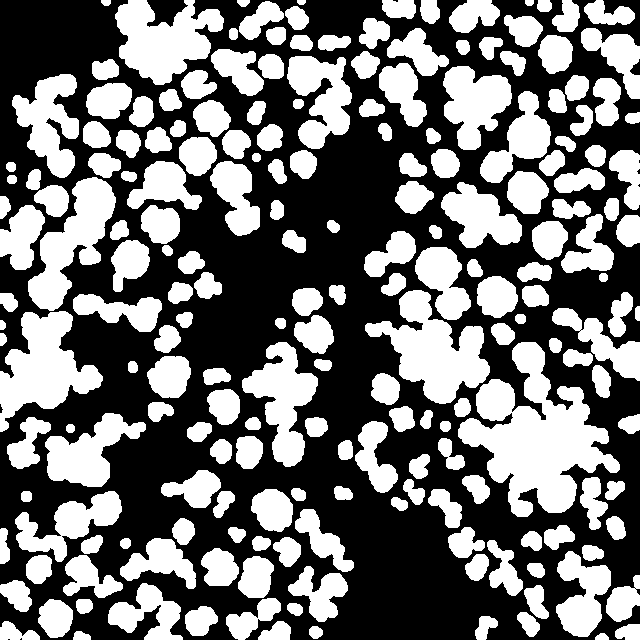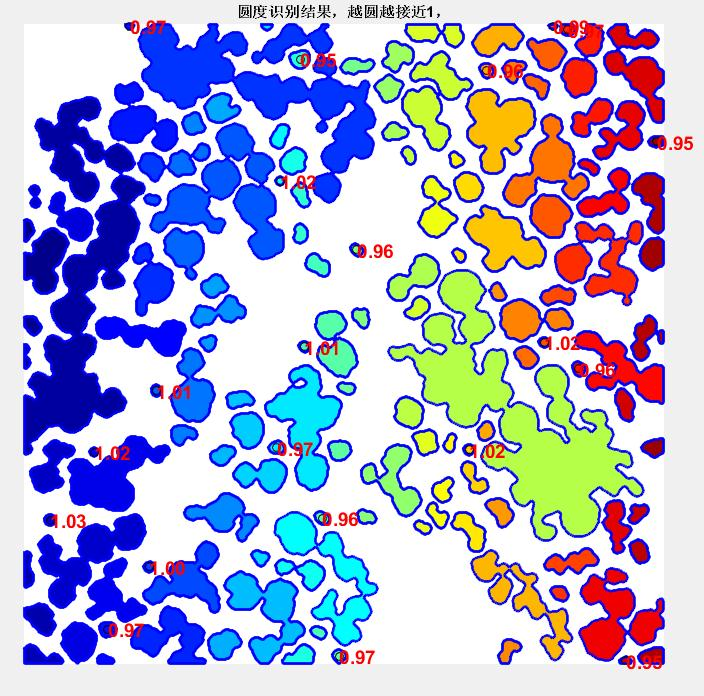2、C++ Opencv
#include<iostream>
#include"opencv2\opencv.hpp"
#include<math.h>

using namespace std;
using namespace cv;

RNG g_rng(12345);
int g_nElementShape=MORPH_RECT;
Mat srcImage, dstImage;

void centerPoints(vector<Point>contour);

int main()
{
srcImage = imread("bw_0133.png", 0);//读取文件，可以是文件目录
if (!srcImage.data){ printf("图片读取错误！\n"); return false; }
namedWindow("原图");
imshow("原图", srcImage);
//进行开运算平滑
//namedWindow("【开运算/闭运算】", 1);
Mat dstImage = Mat::zeros(srcImage.rows, srcImage.cols, CV_8UC3);

Mat element = getStructuringElement(g_nElementShape,
Size(5, 5), Point(-1, -1));
morphologyEx(srcImage, dstImage, MORPH_OPEN, element, Point(-1, -1),2);
imshow("【开运算/闭运算】", dstImage);

vector<vector<Point>>contour;//用来储存轮廓
vector<Vec4i>hierarchy;

findContours(dstImage, contour, hierarchy, RETR_EXTERNAL, CHAIN_APPROX_SIMPLE);

for (unsigned int i = 0; i < contour.size(); ++i)
{
centerPoints(contour[i])；
}

Mat drawing = Mat::zeros(dstImage.size(), CV_8UC3);
for (int unsigned i = 0; i < contour.size(); i++)
{
Scalar color = Scalar(g_rng.uniform(0, 255), g_rng.uniform(0, 255), g_rng.uniform(0, 255));
drawContours(drawing, contour, i, color, 1, 8, vector<Vec4i>(), 0, Point());

}
imshow("轮廓图", drawing);//画出轮廓线,在轮廓线上显示坐标
//计算矩
vector<Moments>mu(contour.size());
for (unsigned int i = 0; i < contour.size(); i++)
{
mu[i] = moments(contour[i], false);
}
//计算矩中心
vector<Point2f>mc(contour.size());
for (unsigned int i = 0; i < contour.size(); i++)
{
mc[i] = Point2f(static_cast<float>(mu[i].m10 / mu[i].m00), static_cast<float>(mu[i].m01 / mu[i].m00));
}
for (unsigned int i = 0; i< contour.size(); ++i)
{
circle(drawing, mc[i], 5, Scalar(0, 0, 255), -1, 8, 0);
rectangle(drawing, boundingRect(contour.at(i)), Scalar(0, 255, 0));
char tam;
sprintf_s(tam, "(%0.0f,%0.0f)", mc[i].x, mc[i].y);
putText(drawing, tam, Point(mc[i].x, mc[i].y), FONT_HERSHEY_SIMPLEX, 0.4, Scalar(255, 0, 255), 1);
//计算质心 坐标
cout << "质心点坐标:" << "(" << (int)mc[i].x << "." << (int)mc[i].y << ")" << endl;
//下标输出
}
namedWindow("Contours", WINDOW_AUTOSIZE);
imshow("Contours", drawing);
waitKey(0);
return 0;
}
void centerPoints(vector<Point>contour)
{
double factor = (contourArea(contour) * 4 * CV_PI) /
(pow(arcLength(contour, true), 2));
cout << "factor:" << factor << endl;  //计算出圆形度factor
}

3、参考
1、matlab 求图像的连通区域的圆度并可视化
https://blog.csdn.net/u012526003/article/details/52522745
2、opencv基础之测试质心和圆形度
https://blog.csdn.net/u014003644/article/details/77779661?utm_source=blogxgwz6

写于关雎新浪微博：https://weibo.com/tianzhejia
CSDN博客：https://blog.csdn.net/qq_35605018
博客网站：http://www.zhijiadeboke.xyz
GitHub：https://github.com/ZhijiaTian
QQ邮箱：2461824656@qq.com
126邮箱：tianzhejia@126.com
Outlook邮箱：tianzhejia@outlook.com
以上均可与本人取得联系，欢迎探讨。^ v ^


展开全文opencv c++ 程序人生 经验分享
• 连通区域的性质 描述 计算二元图像联通区域的统计性质 为了跟踪一个点集合： 建立 vision.BlobAnalysis 对象，并且设置其属性。 像函数一样调用对象，带上参数。 想要知道系统对象如何工作，可以参考 什么是系统...
连通区域的性质
描述
计算二元图像联通区域的统计性质
为了跟踪一个点集合：

建立 vision.BlobAnalysis 对象，并且设置其属性。
像函数一样调用对象，带上参数。
想要知道系统对象如何工作，可以参考 什么是系统对象？

建立
语法
Hblob = vision.BlobAnalysis
Hblob = vision.BlobAnalysis(Name, Value)

描述
Hblob = vision.BlobAnalysis 返回一个区块分析对象，Hblob 被用于计算二元图像连通区域的统计量。

原文这个地方写了个 H，我能报 bug 么？

Hblob = vision.BlobAnalysis(Name, Value) 用一个或多个名称-值对设置属性。每一个属性名称要用引号括起来。例如 Hblob = vision.BlobAnalysis('AreaOutputPort', true)
属性
除非特别声明，属性是 不可以调节的，意味着你在调用对象之后，这些值是不可以改变的。你在调用对象时，这些对象会被锁定，release 函数可以将他们解锁。
如果一个属性是 可以调节的，则你可以在任何时候改变它的值。
改变属性值的更多信息，可以参考 MATLAB 中使用系统对象的系统设计（MATLAB）。

AreaOutputPort — 返回块面积
CentroidOutputPort — 返回块重心坐标
BoundingBoxOutputPort — 返回边界框坐标
MajorAxisLengthOutputPort — 返回椭圆主轴长度向量
MinorAxisLengthOutputPort — 返回椭圆副轴长度向量
OrientationOutputPort — 返回椭圆主轴与 X-轴之间的夹角向量
EccentricityOutputPort — 返回椭圆偏心率向量
EquivalentDiameterSquaredOutputPort — 返回等效直径平方向量
ExtentOutputPort — 返回块面积除以边界框的商向量
PerimeterOutputPort — 返回块周长的估计向量
OutputDataType — 返回统计值的数据类型
Connectivity — 连接像素
LabelMatrixOutputPort — 每一个图片最大标记区域数量
MinimumBlobArea — 最小块面积，以像素为单位
MaximumBlobArea — 最大块面积，以像素为单位
ExcludeBorderBlobs — 去除至少含有一条图像边缘的块
MaximumCount — 每一幅图片最大标记区域数量

什么鬼？为什么这和上面的 LabelMatrixOutputPort 一样 ？

定点数属性

RoundingMethod — 取整方法
OverflowAction — 如果整数超出范围的应对方法
ProductDataType — 乘法数据类型
CustomProductDataType — word 和小数长度
AccumulatorDataType — 累计器数据类型
CustomAccumulatorDataType — 累计 word 和小数长度

用法
语法
[area,centrioid,bbox] = Hblob(bw)
[___,majoraxis] = Hblob(bw)
[___,minoraxis] = Hblob(bw)
[___,orientation] = Hblob(bw)


原文这里是 [bw__, orientation] = Hblob(bw)，我要报 bug 啊

[___,eccentricity] = Hblob(bw)
[___,EQDIASQ] = Hblob(bw)
[___,EXTENT] = Hblob(bw)
[___,perimeter] = Hblob(bw)
[___,label] = Hblob(bw)

描述
输入参数
输出参数
Under Construction…


展开全文• 题目面积与周长的计算 检测下图细胞数量并计算面积与周长的比 处理过程 首先将图像变换为灰度图像并取其边缘二值图像 然后分别对边缘二值掩膜进行膨胀填充空洞清除边缘 最后对处理后的二值图像的连通区域进行检测 求...
• ## MATLAB图形图像处理

热门讨论 2011-01-03 12:20:11
16.2.2 连通对象标注 16.2.3 对象选择 16.2.4 二值图像面积提取 16.2.5 二值图像的欧拉数 16.2.6 移除对象 16.2.7 区域填充 16.3 查找表操作 16.4 灰度形态学 16.4.1 灰度形态学基本运算实例 16.4.2 其它...
• 16.2.2 连通对象标注 16.2.3 对象选择 16.2.4 二值图像面积提取 16.2.5 二值图像的欧拉数 16.2.6 移除对象 16.2.7 区域填充 16.3 查找表操作 16.4 灰度形态学 16.4.1 灰度形态学基本运算实例 16.4.2 其它...
• 文章《（来点有用的）MATLAB基于形态学的目标检测（一）简单图形统计》的演示代码。在一些例如裂纹测量、细胞...直接使用区域增长和连通分量计算，往往结果不好。而通过基本形态学的操作能在一定程度上解决这个问题。
• 16.2.2 连通对象标注 16.2.3 对象选择 16.2.4 二值图像面积提取 16.2.5 二值图像的欧拉数 16.2.6 移除对象 16.2.7 区域填充 16.3 查找表操作 16.4 灰度形态学 16.4.1 灰度形态学基本运算实例 16.4.2 其它...
• 16.2.2 连通对象标注 16.2.3 对象选择 16.2.4 二值图像面积提取 16.2.5 二值图像的欧拉数 16.2.6 移除对象 16.2.7 区域填充 16.3 查找表操作 16.4 灰度形态学 16.4.1 灰度形态学基本运算实例 16.4.2 其它...
• 分水岭变换将图像视为浅色像素代表高海拔而深色像素代表低海拔的表面，从而在图像中找到“集水盆地”或“分水岭脊...指定在分水岭计算中使用的连通性。 发电恢复 说过的在这里插入代码片 close all; clear all; clc; I
分水岭变换将图像视为浅色像素代表高海拔而深色像素代表低海拔的表面，从而在图像中找到“集水盆地”或“分水岭脊线”。 分水岭变换可用于将感兴趣的连续区域分割为不同的对象。
MATLAB图像处理主要是用到了watershed函数使用方法。
调用格式：
L = watershed(A)
返回一个标签矩阵L，该标签矩阵L标识输入矩阵A的分水岭区域。
L = watershed(A,conn)
指定在分水岭计算中使用的连通性。
发电恢复
说过的在这里插入代码片
close all; clear all; clc;
I=imread('circbw.tif');
J=watershed(I,8);   %分水岭分割
figure;
subplot(121);imshow(I);
subplot(122);imshow(J);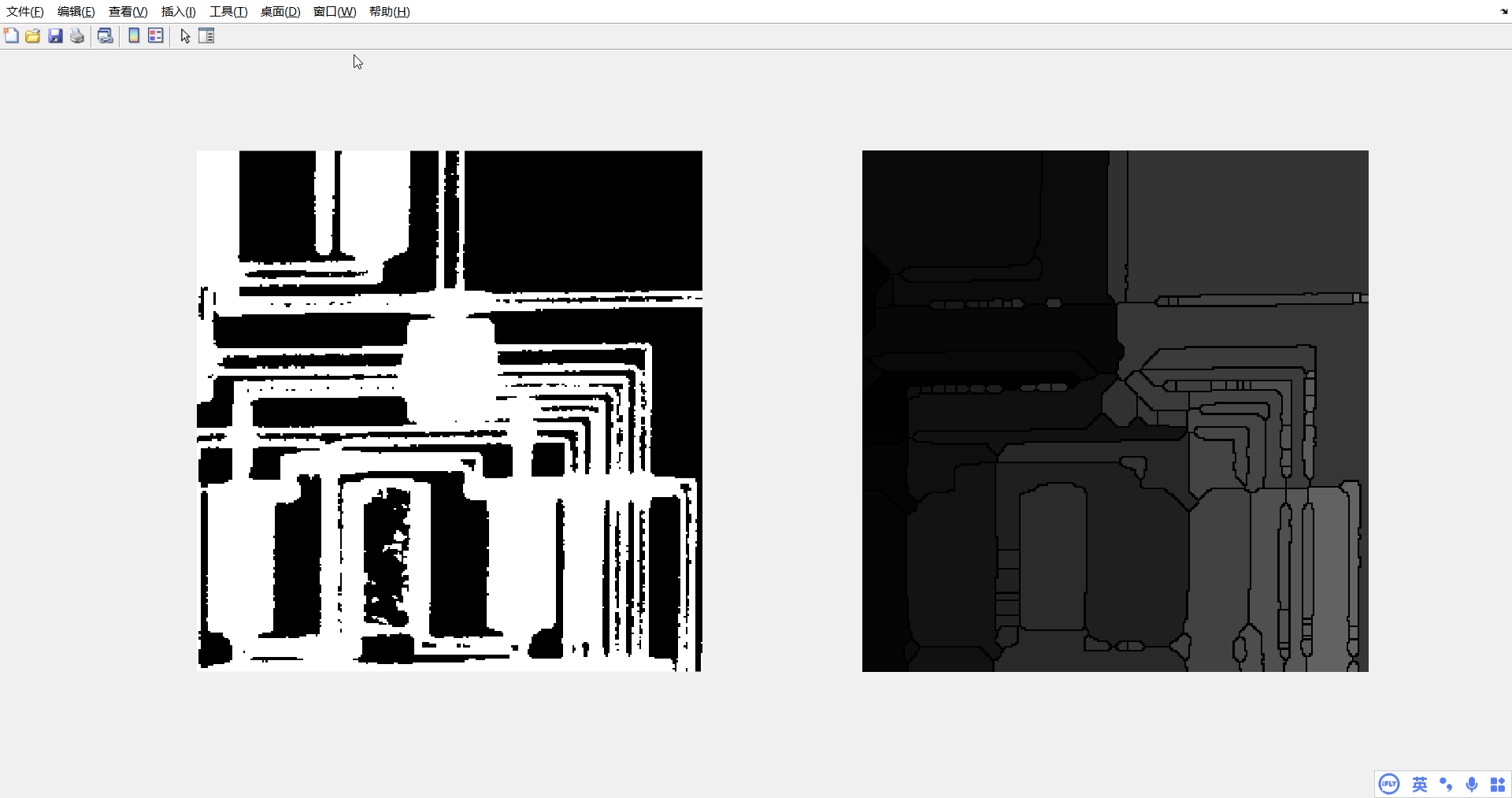`
展开全文算法 计算机视觉
• （2）车牌定位：首先对车牌的二值图片进行形态学滤波，使车牌区域形成一个连通区域，然后根据车牌的先验知识对所得到的连通区域进行筛选，获取车牌区域的具体位置，完成从图片中提取车牌的任务。 （3）车牌分割：...
• 包括 【 直方图均衡化 连通区域面积的计算 均值滤波 中值滤波 高斯平滑模板 NL_means 区域生长 凸包（这个是VC的) Canny 边缘检测 K_means(这个也是VC的哦) 不调用库函数，自己实现的。（额，不是“自己实现”，是...
• 16.2.2 连通对象标注 16.2.3 对象选择 16.2.4 二值图像面积提取 16.2.5 二值图像的欧拉数 16.2.6 移除对象 16.2.7 区域填充 16.3 查找表操作 16.4 灰度形态学 16.4.1 灰度形态学基本运算实例 ...
• ## 超像素分割算法SLIC的matlab实现

千次阅读 多人点赞 2020-08-20 16:59:05
SLIC是一种基于网格化KMeans聚类的超像素分割算法，其计算复杂度为O(N)，其中N为像素点个数。SLIC的原理比较简单精致，具体的原理我这里就不介绍了，推荐大家自己去读原始论文加深理解。SLIC的算法流程如下： 如...
• 基于MATLAB的人脸识别系统： 基于几何特征的算法，对静态人脸从图像采集、预处理、到特征点定位提取，校验通过；主要利用YCbCr肤色模型，通过连通分量提取算法标定人脸五官；对RGB图像通过形态学图像处理算法选定...
• 2. 计算对象面积 3.根据面积大小提取对象 4.查找联通对象 5. 凸壳 6.获得二值图像的边缘图 7.根据属性从二值图像提取对象 8.选择二值图中的对象 9.标记连通分量 10.创建标签矩阵 一、对象分析 ...
• 在一些例如裂纹测量、细胞统计的工作中，由于目标可能连接、重叠等情况。直接使用区域增长和连通分量计算，往往结果不好。而通过基本形态学的操作能在一定程度上解决这个问题。本文分享在简单目标上的运用方法。目标检测
• ②车牌定位：首先对车牌的二值图片进行形态学滤波，使车牌区域形成一个连通区域，然后根据车牌的先验知识对所得到的连通区域进行筛选，获取车牌区域的具体位置，完成从图片中提取车牌的任务。 ③车牌分割：首先对...
• <p>%% 选择出剩下连通区域中面积最大的区域，去除其他干扰 tL=L.*0; aera=zeros(num,1); for i=1:num  tL=L.*0;  tL(L==i)=1;  aera(i)&...
• 下面以一幅被遮挡的图I为例进行代码的详细分析：1、 灰度图的二值化，计算连通区域输入的灰度图为I，显示如下 二值化方法是基于Peter Kovesi在http://www.csse.uwa.edu.au/~pk/Research/MatlabFns/提供的adap源代码 代码解析
• 目录 1、数字图像的构成 2、不同环境图像的存储方式 ...5.1、邻接性、连通性、区域和边界 5.1.1 邻接性 5.1.2、连通性 5.1.3、区域和边界 5.1.4、度量图像的距离 5.2、图像的基本操作 5.2....Image 数字图像处理# matlab计算连通区域matlab 订阅